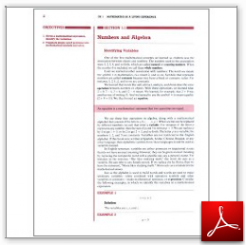Algebra Math Book - Introductory Algebra Thank you for your continual support.

 The power of mathematics rests in the logic of thinking.ID: Sec1-1
Description: Identifying Variables-Translating Word Problems-Grouping Symbols
Price: .99

# Algebra Math book - Introductory Algebra - Chapter 1 - Section 1 - Numbers and Algebra

## Section 1.1 - Objectives

1.  Given a mathematical expression, identify the variables.

2.  Translate simple word problems into mathematical symbols and solve.

Navigate to
Previous Page or Next Section
Other Chapters

For a more detailed explanation please visit:  Numbers and Algebra

This section of my algebra math book, Introductory Algebra, also includes in the download:

• Cover Sheet